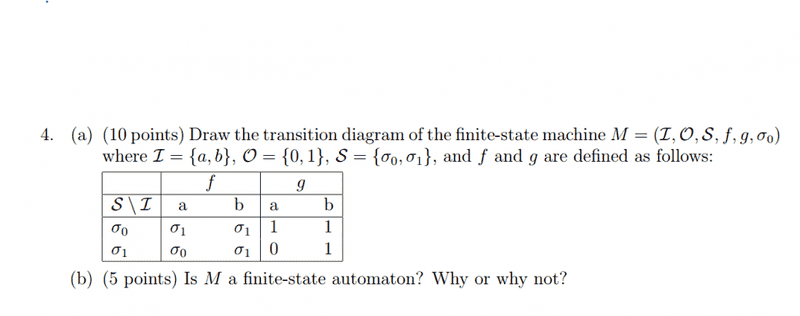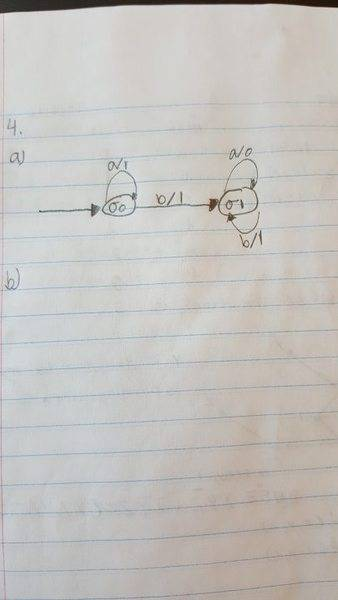# Transition Diagrams and finite-state automaton?

• Kingyou123

## Homework Statement## Homework Equations

3. The Attempt at a Solution [/B]My confusion comes in with b/1, would it be going back to sigma inital since b/1=1?
Also could someone explain what a finite state automaton is.

I'm not familiar with the table format given, but it seems self-explanatory. If I understand it, I disagree with your answer diagram. Tell me how you interpret the σ1 directly under the a in the f column. I.e., in the row with σ0 in the left hand column.

I'm not familiar with the table format given, but it seems self-explanatory. If I understand it, I disagree with your answer diagram. Tell me how you interpret the σ1 directly under the a in the f column. I.e., in the row with σ0 in the left hand column.
σ1 is when the output is a =1 and b=1

σ1 is when the output is a =1 and b=1
I think you completely misunderstand the table. The sigmas are the states, old and new. a and b are the values of the input (it's either an a or a b, not a 0 or a 1), and the 0 and 1 are the outputs. The functions f and g are the state change function and the output function respectively.

The first row says that in state σ0 an input of a leads to an output of 1 and a new state σ1; an input of b does the same.
The second row says that in state σ1 an input of a leads to an output of 0 and a new state σ0; an input of b does the same as it does in state σ0.